Anúncio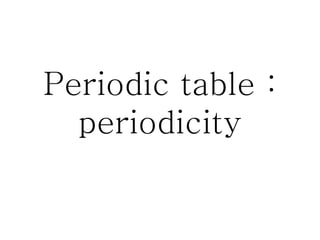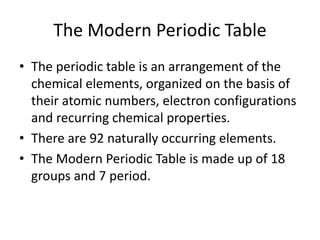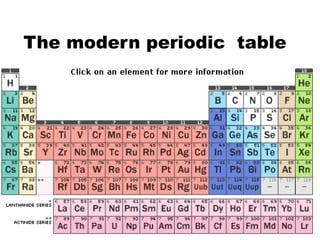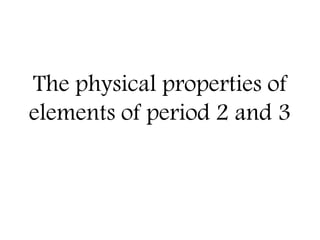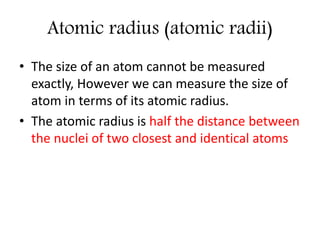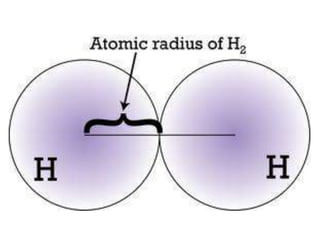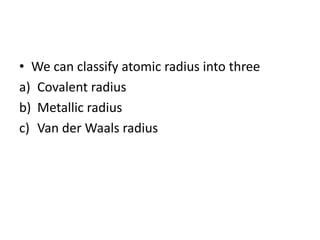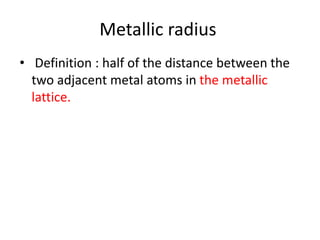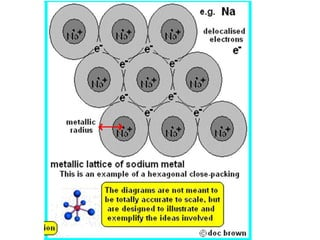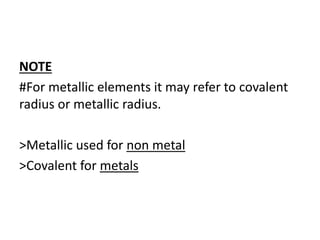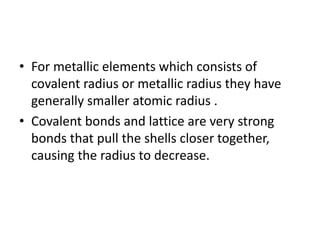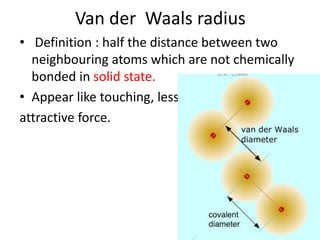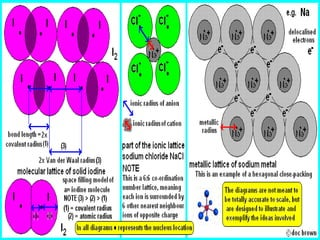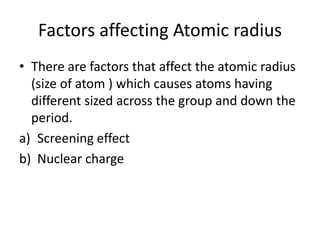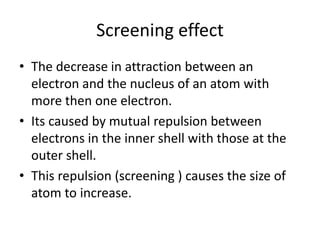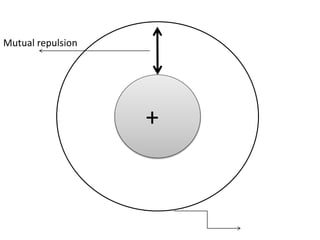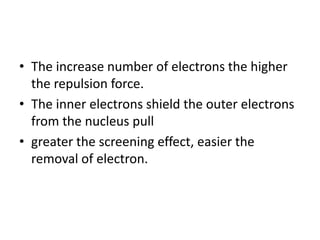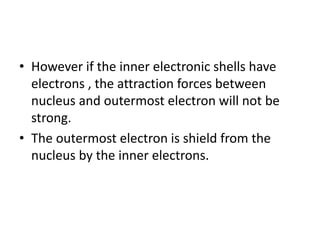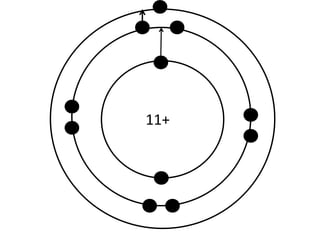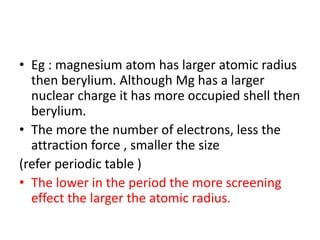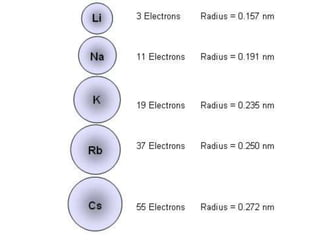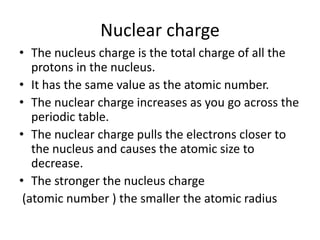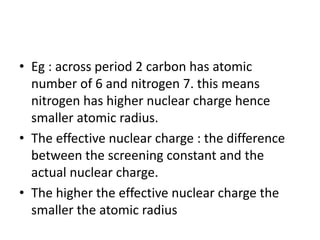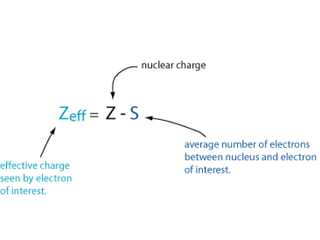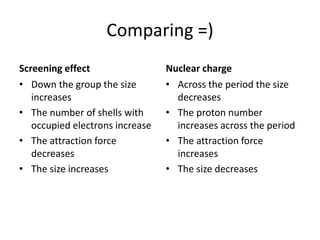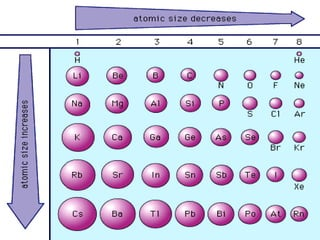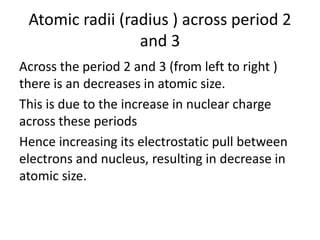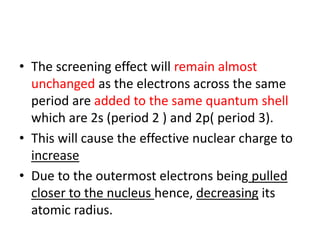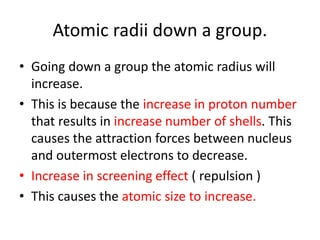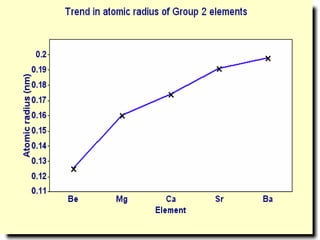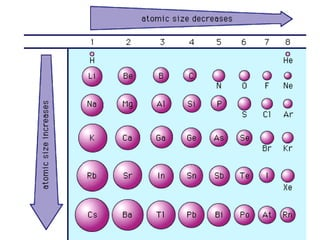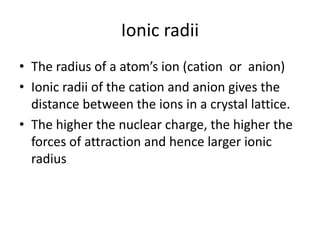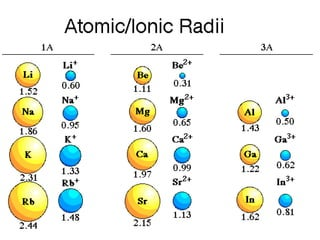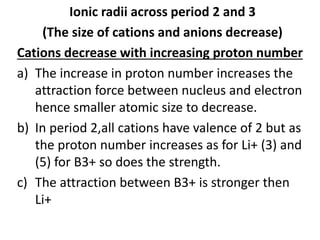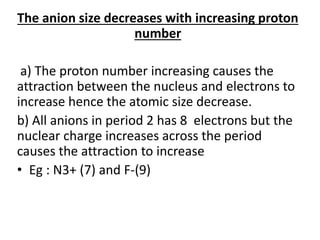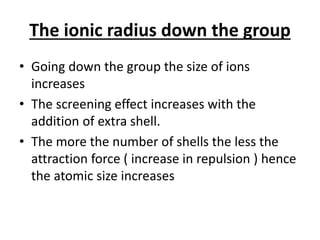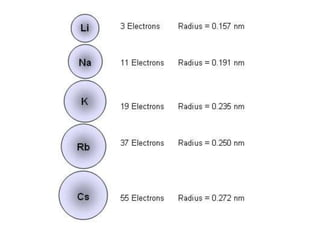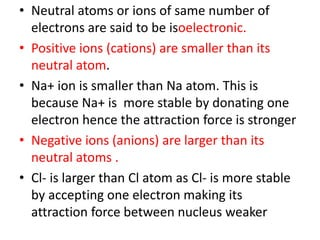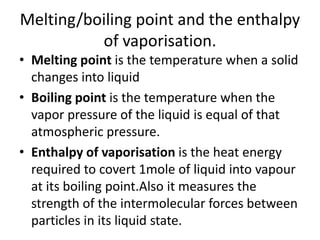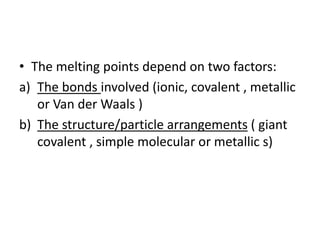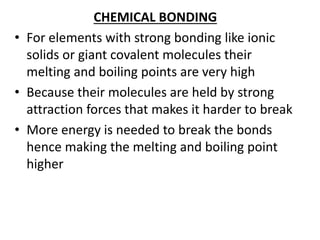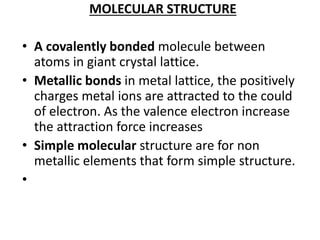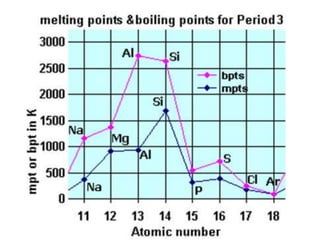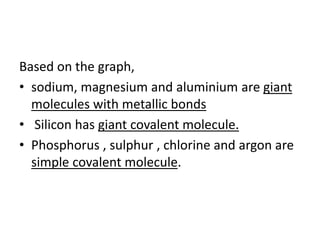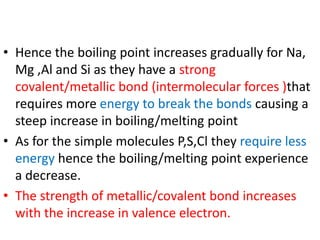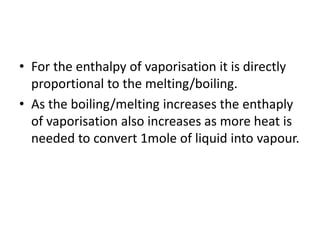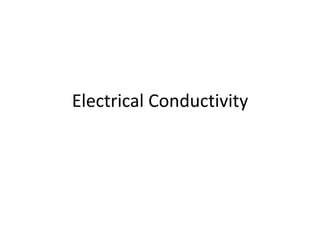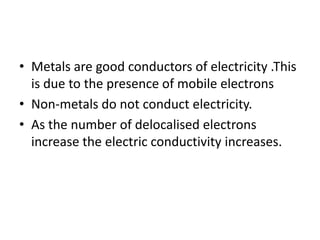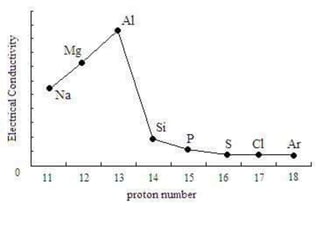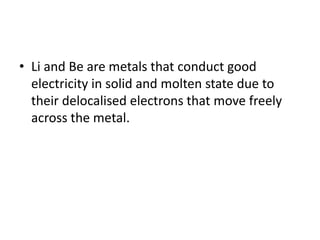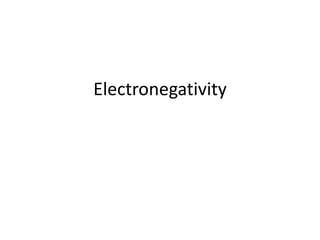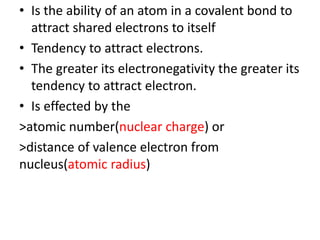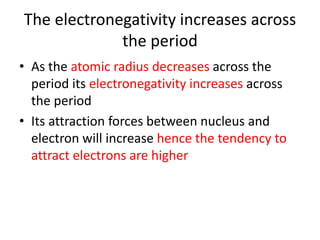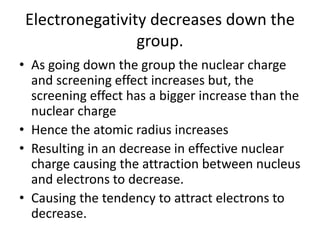1 de 55
Anúncio

### Inorganic Chemistry: Period 2 and 3

1. Periodic table : periodicity
2. The Modern Periodic Table • The periodic table is an arrangement of the chemical elements, organized on the basis of their atomic numbers, electron configurations and recurring chemical properties. • There are 92 naturally occurring elements. • The Modern Periodic Table is made up of 18 groups and 7 period.
3. The modern periodic table
4. The physical properties of elements of period 2 and 3
5. Atomic radius (atomic radii) • The size of an atom cannot be measured exactly, However we can measure the size of atom in terms of its atomic radius. • The atomic radius is half the distance between the nuclei of two closest and identical atoms
7. Covalent radius • Definition : half the length from one nuclei to another atom’s nuclei bonded covalently.
8. Metallic radius • Definition : half of the distance between the two adjacent metal atoms in the metallic lattice.
9. NOTE #For metallic elements it may refer to covalent radius or metallic radius. >Metallic used for non metal >Covalent for metals
10. • For metallic elements which consists of covalent radius or metallic radius they have generally smaller atomic radius . • Covalent bonds and lattice are very strong bonds that pull the shells closer together, causing the radius to decrease.
11. Van der Waals radius • Definition : half the distance between two neighbouring atoms which are not chemically bonded in solid state. • Appear like touching, less attractive force.
12. Factors affecting Atomic radius • There are factors that affect the atomic radius (size of atom ) which causes atoms having different sized across the group and down the period. a) Screening effect b) Nuclear charge
13. Screening effect • The decrease in attraction between an electron and the nucleus of an atom with more then one electron. • Its caused by mutual repulsion between electrons in the inner shell with those at the outer shell. • This repulsion (screening ) causes the size of atom to increase.
14. + Mutual repulsion
15. • The increase number of electrons the higher the repulsion force. • The inner electrons shield the outer electrons from the nucleus pull • greater the screening effect, easier the removal of electron.
16. • However if the inner electronic shells have electrons , the attraction forces between nucleus and outermost electron will not be strong. • The outermost electron is shield from the nucleus by the inner electrons.
17. 11+
18. • Eg : magnesium atom has larger atomic radius then berylium. Although Mg has a larger nuclear charge it has more occupied shell then berylium. • The more the number of electrons, less the attraction force , smaller the size (refer periodic table ) • The lower in the period the more screening effect the larger the atomic radius.
19. Nuclear charge • The nucleus charge is the total charge of all the protons in the nucleus. • It has the same value as the atomic number. • The nuclear charge increases as you go across the periodic table. • The nuclear charge pulls the electrons closer to the nucleus and causes the atomic size to decrease. • The stronger the nucleus charge (atomic number ) the smaller the atomic radius
20. • Eg : across period 2 carbon has atomic number of 6 and nitrogen 7. this means nitrogen has higher nuclear charge hence smaller atomic radius. • The effective nuclear charge : the difference between the screening constant and the actual nuclear charge. • The higher the effective nuclear charge the smaller the atomic radius
21. Comparing =) Screening effect • Down the group the size increases • The number of shells with occupied electrons increase • The attraction force decreases • The size increases Nuclear charge • Across the period the size decreases • The proton number increases across the period • The attraction force increases • The size decreases
22. Atomic radii (radius ) across period 2 and 3 Across the period 2 and 3 (from left to right ) there is an decreases in atomic size. This is due to the increase in nuclear charge across these periods Hence increasing its electrostatic pull between electrons and nucleus, resulting in decrease in atomic size.
23. • The screening effect will remain almost unchanged as the electrons across the same period are added to the same quantum shell which are 2s (period 2 ) and 2p( period 3). • This will cause the effective nuclear charge to increase • Due to the outermost electrons being pulled closer to the nucleus hence, decreasing its atomic radius.
24. Atomic radii down a group. • Going down a group the atomic radius will increase. • This is because the increase in proton number that results in increase number of shells. This causes the attraction forces between nucleus and outermost electrons to decrease. • Increase in screening effect ( repulsion ) • This causes the atomic size to increase.
25. Ionic radii • The radius of a atom’s ion (cation or anion) • Ionic radii of the cation and anion gives the distance between the ions in a crystal lattice. • The higher the nuclear charge, the higher the forces of attraction and hence larger ionic radius
26. Ionic radii across period 2 and 3 (The size of cations and anions decrease) Cations decrease with increasing proton number a) The increase in proton number increases the attraction force between nucleus and electron hence smaller atomic size to decrease. b) In period 2,all cations have valence of 2 but as the proton number increases as for Li+ (3) and (5) for B3+ so does the strength. c) The attraction between B3+ is stronger then Li+
27. The anion size decreases with increasing proton number a) The proton number increasing causes the attraction between the nucleus and electrons to increase hence the atomic size decrease. b) All anions in period 2 has 8 electrons but the nuclear charge increases across the period causes the attraction to increase • Eg : N3+ (7) and F-(9)
28. The ionic radius down the group • Going down the group the size of ions increases • The screening effect increases with the addition of extra shell. • The more the number of shells the less the attraction force ( increase in repulsion ) hence the atomic size increases
29. • Neutral atoms or ions of same number of electrons are said to be isoelectronic. • Positive ions (cations) are smaller than its neutral atom. • Na+ ion is smaller than Na atom. This is because Na+ is more stable by donating one electron hence the attraction force is stronger • Negative ions (anions) are larger than its neutral atoms . • Cl- is larger than Cl atom as Cl- is more stable by accepting one electron making its attraction force between nucleus weaker
30. Melting/boiling point and the enthalpy of vaporisation. • Melting point is the temperature when a solid changes into liquid • Boiling point is the temperature when the vapor pressure of the liquid is equal of that atmospheric pressure. • Enthalpy of vaporisation is the heat energy required to covert 1mole of liquid into vapour at its boiling point.Also it measures the strength of the intermolecular forces between particles in its liquid state.
31. • The melting points depend on two factors: a) The bonds involved (ionic, covalent , metallic or Van der Waals ) b) The structure/particle arrangements ( giant covalent , simple molecular or metallic s)
32. CHEMICAL BONDING • For elements with strong bonding like ionic solids or giant covalent molecules their melting and boiling points are very high • Because their molecules are held by strong attraction forces that makes it harder to break • More energy is needed to break the bonds hence making the melting and boiling point higher
33. MOLECULAR STRUCTURE • A covalently bonded molecule between atoms in giant crystal lattice. • Metallic bonds in metal lattice, the positively charges metal ions are attracted to the could of electron. As the valence electron increase the attraction force increases • Simple molecular structure are for non metallic elements that form simple structure. •
34. Based on the graph, • sodium, magnesium and aluminium are giant molecules with metallic bonds • Silicon has giant covalent molecule. • Phosphorus , sulphur , chlorine and argon are simple covalent molecule.
35. • Hence the boiling point increases gradually for Na, Mg ,Al and Si as they have a strong covalent/metallic bond (intermolecular forces )that requires more energy to break the bonds causing a steep increase in boiling/melting point • As for the simple molecules P,S,Cl they require less energy hence the boiling/melting point experience a decrease. • The strength of metallic/covalent bond increases with the increase in valence electron.
36. • For the enthalpy of vaporisation it is directly proportional to the melting/boiling. • As the boiling/melting increases the enthaply of vaporisation also increases as more heat is needed to convert 1mole of liquid into vapour.
37. Electrical Conductivity
38. • Metals are good conductors of electricity .This is due to the presence of mobile electrons • Non-metals do not conduct electricity. • As the number of delocalised electrons increase the electric conductivity increases.
39. • Li and Be are metals that conduct good electricity in solid and molten state due to their delocalised electrons that move freely across the metal.
40. Electronegativity
41. • Is the ability of an atom in a covalent bond to attract shared electrons to itself • Tendency to attract electrons. • The greater its electronegativity the greater its tendency to attract electron. • Is effected by the >atomic number(nuclear charge) or >distance of valence electron from nucleus(atomic radius)
42. The electronegativity increases across the period • As the atomic radius decreases across the period its electronegativity increases across the period • Its attraction forces between nucleus and electron will increase hence the tendency to attract electrons are higher
43. Electronegativity decreases down the group. • As going down the group the nuclear charge and screening effect increases but, the screening effect has a bigger increase than the nuclear charge • Hence the atomic radius increases • Resulting in an decrease in effective nuclear charge causing the attraction between nucleus and electrons to decrease. • Causing the tendency to attract electrons to decrease.
Anúncio